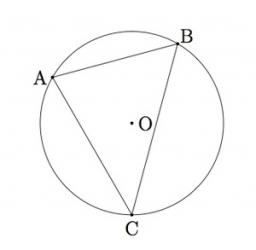# Triangle 3552

Draw a circle k (S, r = 3cm). Build a triangle ABC so that its vertices lie on the circle k and the length of the sides is (AB) = 2.5 cm (AC) = 4 cm

a =  4.175 cm

### Step-by-step explanation:

Try calculation via our triangle calculator.Did you find an error or inaccuracy? Feel free to write us. Thank you!

Tips for related online calculators
Do you want to convert length units?

#### Grade of the word problem:

We encourage you to watch this tutorial video on this math problem: